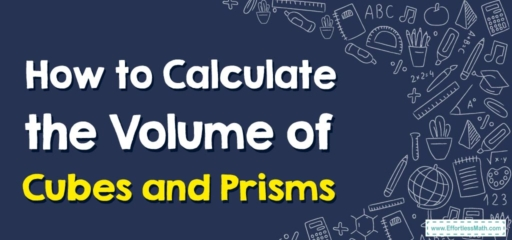# How to Calculate the Volume of Cubes and Prisms

Today, we're taking a deep dive into the third dimension with cubes and prisms, exploring how to calculate their volume.## 1. Understanding Volume

Volume refers to the amount of space that a three-dimensional object occupies. It’s measured in cubic units (like cubic inches, cubic feet, or cubic centimeters).

## 2. Calculating the Volume of Cubes and Prisms

Calculating volume depends on the shape of the object. For cubes and prisms (including rectangular prisms), it involves multiplying the area of the base by the height.

### Step-By-Step Guide to Calculating the Volume of Cubes and Prisms

Let’s break down the process:

#### Step 1: Identify the Base and the Height

The base is the bottom face of the prism, and the height is the distance from the base to the top of the prism. In a cube, all faces are identical, so any face can be considered the base.

#### Step 2: Calculate the Area of the Base

For a cube, since all sides are of equal length (say, $$s$$), the area of the base is $$s^2$$. For a rectangular prism, the area of the base is $$length\times width$$.

#### Step 3: Multiply the Area of the Base by the Height

This will give you the volume of the cube or prism. So, $$Volume = base area\times height$$.

For example, if you have a cube with a side length of $$3$$ units:

1. Identify the base and height: The base is a square with a side length of $$3$$ units, and the height is also $$3$$ units.
2. Calculate the area of the base: The area is $$3^2 = 9$$ square units.
3. Multiply the area of the base by the height: The volume is $$9\times 3 = 27$$ cubic units.

And there you have it! That’s how you calculate the volume of cubes and prisms.
Keep practicing, keep exploring, and enjoy your mathematical journey!

In this blog post, we’ve explained how to calculate the volume of cubes and prisms. We also provided a step-by-step guide to make this process easy to understand and implement. With practice, you’ll master this essential geometric skill in no time. Happy calculating!

### What people say about "How to Calculate the Volume of Cubes and Prisms - Effortless Math: We Help Students Learn to LOVE Mathematics"?

No one replied yet.

X
30% OFF

Limited time only!

Save Over 30%

SAVE $5 It was$16.99 now it is \$11.99